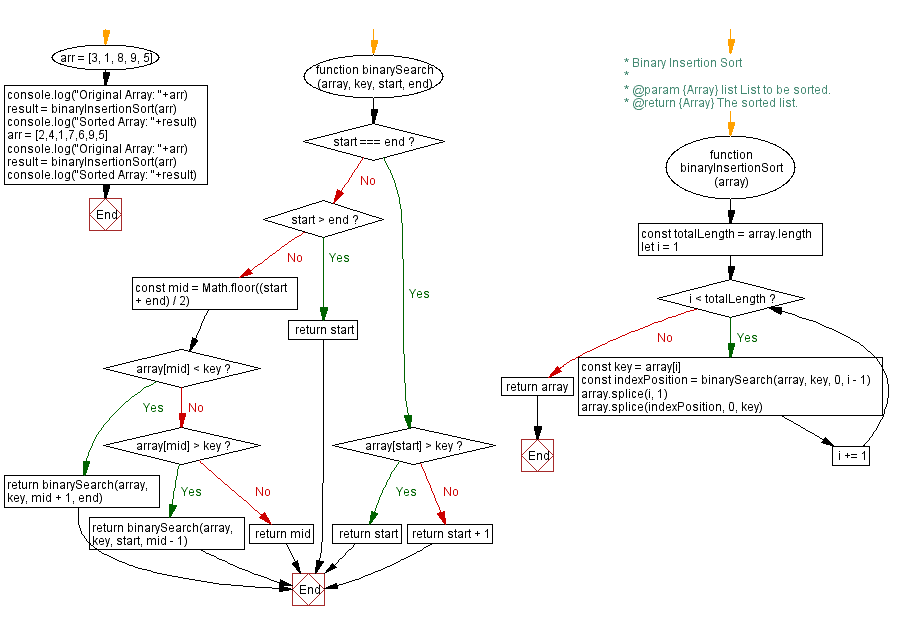# JavaScript Sorting Algorithm: Binary Insertion sort

## JavaScript Sorting Algorithm: Exercise-27 with Solution

From Wikipedia,
Binary insertion sort employs a binary search to determine the correct location to insert new elements, and therefore performs [log2 n] comparisons in the worst case. When each element in the array is searched for and inserted this is O(n log n). The algorithm as a whole still has a running time of O(n2) on average because of the series of swaps required for each insertion.

Write a JavaScript program to sort a list of elements using the Binary Insertion sort algorithm.

Sample Data: Original Array: [3, 1, 8, 9, 5]
Sorted Array: [1, 3, 5, 8, 9]
Original Array: [2, 4, 1, 7, 6, 9, 5]
Sorted Array: [1, 2, 4, 5, 6, 7, 9]

Sample Solution:

JavaScript Code:

``````/**
* @param {Array} array Array of numbers.
* @param {Number} key Value to be searched
* @param {Number} start start index position of array
* @param {Number} end end index position of array
* @return {Number} Position of the key element
*/
function binarySearch (array, key, start, end) {
if (start === end) {
if (array[start] > key) {
return start
} else {
return start + 1
}
}

if (start > end) {
return start
}

const mid = Math.floor((start + end) / 2)

if (array[mid] < key) {
return binarySearch(array, key, mid + 1, end)
} else if (array[mid] > key) {
return binarySearch(array, key, start, mid - 1)
} else {
return mid
}
}

/**
* Binary Insertion Sort
*
* @param {Array} list List to be sorted.
* @return {Array} The sorted list.
*/
function binaryInsertionSort (array) {
const totalLength = array.length
for (let i = 1; i < totalLength; i += 1) {
const key = array[i]
const indexPosition = binarySearch(array, key, 0, i - 1)
array.splice(i, 1)
array.splice(indexPosition, 0, key)
}
return array
}
arr = [3, 1, 8, 9, 5]
console.log("Original Array: "+arr)
result = binaryInsertionSort(arr)
console.log("Sorted Array: "+result)
arr = [2,4,1,7,6,9,5]
console.log("Original Array: "+arr)
result = binaryInsertionSort(arr)
console.log("Sorted Array: "+result)
```
```

Sample Output:

```"Original Array: 3,1,8,9,5"
"Sorted Array: 1,3,5,8,9"
"Original Array: 2,4,1,7,6,9,5"
"Sorted Array: 1,2,4,5,6,7,9"
"Original Array: 3,1,8,9,5"
"Sorted Array: 1,3,5,8,9"
"Original Array: 2,4,1,7,6,9,5"
"Sorted Array: 1,2,4,5,6,7,9"
```

Flowchart:Live Demo:

See the Pen searching-and-sorting-algorithm-exercise-27 by w3resource (@w3resource) on CodePen.

Improve this sample solution and post your code through Disqus

Previous: Alpha Numerical Sort.
Next: Cycle sort.

What is the difficulty level of this exercise?

Test your Programming skills with w3resource's quiz.

﻿